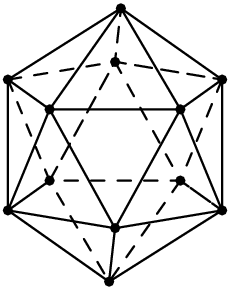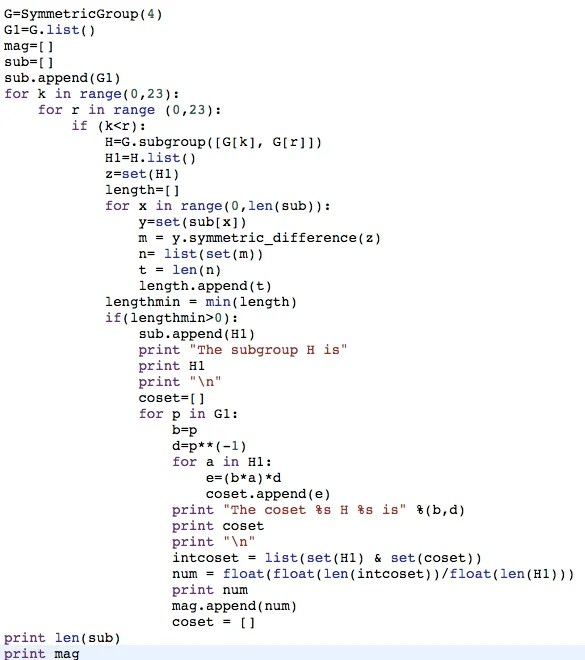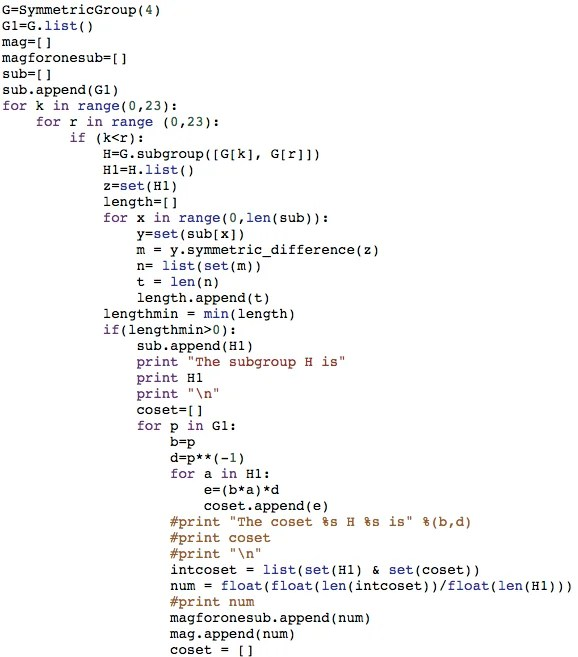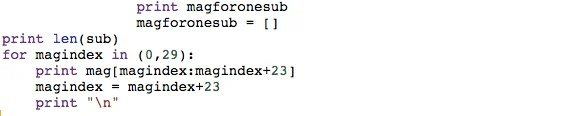## Round robin tournament

Problem : Suppose there are $${k}$$ teams playing a round robin tournament; that is, each team plays against all the other teams and no game ends in a draw.Suppose the $${i^{th}}$$ team loses $${l_{i}}$$ games and wins $${w_{i}}$$ games.Show that

$${{\displaystyle}{\sum_{i=1}^{k}{l_i^{2}}}}$$ = $${{\displaystyle}{\sum_{i=1}^{k}{w_i^{2}}}}$$

Solution : Each team plays exactly one match against each other team.

Consider the expression $$\displaystyle{\sum_{i=1}^{k} l_i^{2} – {w_i^2} = \sum_{i=1}^{k}(l_i + w_i)(l_i – w_i) }$$

Since each team plays exactly k-1 matches and no match ends in a draw, hence number of wins plus numbers of loses of a particular team is k-1 (that is the number of matches it has played). In other words $$l_i + w_i = k-1$$ for all i (from 1 to k).

Hence

$$\displaystyle{\sum_{i=1}^{k} l_i^{2} – {w_i^2} }$$
$$\displaystyle{= \sum_{i=1}^{k}(l_i + w_i)(l_i – w_i) }$$
$$\displaystyle{= \sum_{i=1}^{k}(k-1)(l_i – w_i) }$$
$$\displaystyle{= (k-1)\left( \sum_{i=1}^{k} l_i – \sum_{i=1}^{k} w_i\right) }$$

But $$\displaystyle{ \sum_{i=1}^{k} l_i = \sum_{i=1}^{k} w_i }$$ (as total number of loses = total number of matches = total number of wins; as each match results in a win or lose of some one)

Hence $$\displaystyle{= (k-1)\left( \sum_{i=1}^{k} l_i – \sum_{i=1}^{k} w_i\right) = (k-1) \times 0 = 0 }$$

Therefore $$\displaystyle{\sum_{i=1}^{k} l_i^{2} – {w_i^2} = 0 }$$ implying $${{\displaystyle}{\sum_{i=1}^{k}{l_i^{2}}}}$$ = $${{\displaystyle}{\sum_{i=1}^{k}{w_i^{2}}}}$$

Proved.

## Number Theory 1 Teaching Schedule

This document is useful for current students. It contains teaching schedule for Number Theory 1.

## Overview:

Number Theory 1 is an introductory module. It is useful for beginner math olympiad aspirants (preparing for AMC, AIME, ARML, Duke Math Meet etc.)

• Number systems
• Prime numbers
• Arithmetic and geometric sequences
• Mathematical Induction
• Divisibility techniques
• Arithmetic of remainders
• Modular Arithmetic and Gauss’s theory
• Equivalence Relations
• Mathematical games

## Specifications

• Each session (day) is 2 hours long.
• It is followed by a homework assignment.
• Apart from regular theoretical work and problem-solving, each section consists of mathematical games
• Books:
• Challenges and Thrills of Pre-College Mathematics
• Mathematics can be Fun by Yakov Perelman
• Excursion Into Mathematics
• Mathematical Circles, Russian Experience by Fomin

## Sessions

### Session 1

• Formula for nth odd number and nth even number
• Sum of first n odd numbers and their visual treatment
• Arithmetic Progression

### Session 2 and 3

• Arithmetic Progression’s description (nth number)
• Gauss’s method for summing arithmetic progression (rewriting a finite sum in reverse order).
• Sum of n terms of an arithmetic sequence
• Geometric sequence
• Sum of nth term of a geometric sequence

### Session 4 and 5

• Mathematical induction
• Strong form of induction

### Session 6 and 7

• Divisibility and prime numbers
• Fundamental Theorem of Arithmetic

### Session 8

• Types of numbers
• Well ordering principles
• Irrationality of square root of 2 (and primes)

### Session 9 and 10

• Modular Arithmetic – similarity and differences with equality
• Notion of equivalence relation

## Combinatorics Problem List for AIME

This is a collection of combinatorics and probability problems that have appeared in AIME.

1. Two dice appear to be standard dice with their faces numbered from $$1$$ to $$6$$, but each die is weighted so that the probability of rolling the number $$k$$ is directly proportional to $$k$$. The probability of rolling a $$7$$ with this pair of dice is $$\tfrac{m}{n}$$, where $$m$$ and $$n$$ are relatively prime positive integers. Find $$m + n$$. (2016, AIME I, 2)
2. A regular icosahedron is a $$20$$-faced solid where each face is an equilateral triangle and five triangles meet at every vertex. The regular icosahedron shown below has one vertex at the top, one vertex at the bottom, an upper pentagon of five vertices all adjacent to the top vertex and all in the same horizontal plane, and a lower pentagon of five vertices all adjacent to the bottom vertex and all in another horizontal plane. Find the number of paths from the top vertex to the bottom vertex such that each part of a path goes downward or horizontally along an edge of the icosahedron, and no vertex is repeated. (2016, AIME I, 3)3. For a permutation $$p = (a_1,a_2,\ldots,a_9)$$ of the digits $$1,2,\ldots,9$$, let $$s(p)$$ denote the sum of the three $$3$$-digit numbers $$a_1a_2a_3$$, $$a_4a_5a_6$$, and $$a_7a_8a_9$$. Let $$m$$ be the minimum value of $$s(p)$$ subject to the condition that the units digit of $$s(p)$$ is $$0$$. Let $$n$$ denote the number of permutations $$p$$ with $$s(p) = m$$. Find $$|m – n|$$. (2016, AIME I, 8)
4. Freddy the frog is jumping around the coordinate plane searching for a river, which lies on the horizontal line $$y = 24$$. A fence is located at the horizontal line $$y = 0$$. On each jump Freddy randomly chooses a direction parallel to one of the coordinate axes and moves one unit in that direction. When he is at a point where $$y=0$$, with equal likelihoods he chooses one of three directions where he either jumps parallel to the fence or jumps away from the fence, but he never chooses the direction that would have him cross over the fence to where $$y < 0$$. Freddy starts his search at the point $$(0, 21)$$ and will stop once he reaches a point on the river. Find the expected number of jumps it will take Freddy to reach the river. (2016, AIME I, 13)
5. There is a $$40\%$$ chance of rain on Saturday and a $$30\%$$ of rain on Sunday. However, it is twice as likely to rain on Sunday if it rains on Saturday than if it does not rain on Saturday. The probability that it rains at least one day this weekend is $$\frac{a}{b}$$, where $$a$$ and $$b$$ are relatively prime positive integers. Find $$a+b$$.
6. For positive integers $$N$$ and $$k$$, define $$N$$ to be $$k$$-nice if there exists a positive integer $$a$$ such that $$a^k$$ has exactly $$N$$ positive divisors. Find the number of positive integers less than $$1000$$ that are neither $$7$$-nice nor $$8$$-nice.
7. The figure below shows a ring made of six small sections which you are to paint on a wall. You have four paint colors available and will paint each of the six sections a solid color. Find the number of ways you can choose to paint each of the six sections if no two adjacent section can be painted with the same color.8. Beatrix is going to place six rooks on a $$6\times6$$ chessboard where both the rows and columns are labelled $$1$$ to $$6$$; the rooks are placed so that no two rooks are in the same row or the same column. The value of a square is the sum of its row number and column number. The score of an arrangement of rooks is the least value of any occupied square. The average score over all valid configurations is $$\frac{p}{q}$$, where $$p$$ and $$q$$ are relatively prime positive integers. Find $$p+q$$.

## Construction of polynomials

The polynomial P(x) has the property that P(1), P(2), P(3), P(4), and P(5) are equal to 1, 2, 3, 4, 5 in some order. How many possibilities are there for the polynomial P, given that the degree of P is strictly less than 4?

(Duke Math Meet 2013 Tiebreaker round)

Discussion:

Let $$P(x) = a x^3 + b x^2 + c x + d$$

Suppose P(1), P(2), P(3), P(4) are p,q,r,s respectively. Then in matrix notation we may write:

## A mathematician’s bookshelf

A mathematician’s bookshelf is probably more informative than his resume.

The idea of ‘book’ has been recently challenged by the advent of technology. Outstanding authors such as Hatcher (of ‘Algebraic Topology’ fame) prefers to keep an electronic copy of his book. This electronic copy is updated from time to time.

## Chapter 11 (Rings) – Artin – Section 1 (Definition of a Ring)

1.

Note that a complex number $$\alpha$$ is algebraic if it is a root of a(nonzero) polynomial with integer coefficients.
a) Sine we need $$7 + \sqrt {2}$$ to be root of an integer polynomial, there fore $$(x – (7 + \sqrt {2}) = (x-7 – 2^{\frac{1}{3}})$$ is one of the factors. Clearly $$(x-7)^3 – (\sqrt {2})^3$$ $$= (x-7)^3 – 2$$ is the desired polynomial.
b) Again we need $$\sqrt 3 + \sqrt {-5}$$ as a root of an integer polynomial. Therefore $$(x – (\sqrt 3 + \sqrt {-5}))$$ is one of the factors. Take another factor as $$(x – (\sqrt 3 – \sqrt {-5}))$$ (motivation is to get rid of square roots).
$$(x – (\sqrt 3 + \sqrt {-5})) \times (x – (\sqrt 3 – \sqrt {-5})) = x^2 – 2\sqrt 3 + 8$$
Finally we multiply $$x^2 + 8 + 2\sqrt 3$$ to that to get rid of the final square root.
$$(x^2 – 2\sqrt 3 + 8) \times (x^2 + 8 + 2\sqrt 3) = (x^2 + 8)^2 – 12 x^2$$

## Straight Lines and Curves by Vasiliyev

N. B. Vasilyev was the chief architect of Mathematical Olympiads in Soviet Union. This gem from erstwhile Soviet Union’s publication, explores loci of points in plane and space. The entire discussion is aided by geometric intuition. The authors occasionally use algebraic tools to augment the ideas. The holistic nature of the discussion is truly breathtaking.

## Shrinking the diameter of Vitali Set

Claim: The diameter of Vitali Set V on [0, 1] can be shrinked as much as we please

Proof:

Let R be an equivalence relation defined on [0,1] such that x is related to if x – y is rational.

Let E be an equivalence class corresponding to the equivalence relation R.

In order to create the Vitali Set V (on [0,1] ) we need to pick exactly one element from each such equivalence class.

We will show that for an arbitrary equivalence class E and for any natural number n, it is possible to find an element in such that 1/n

Suppose z’ is in E and 1/n < z’

By Archimedean property we can say that there exists a natural number p such that p/n > z’

Let P be the set of all numbers such that p/n > z’

Now by well ordering principle P has a least element p*.

Hence (p* -1)/n < z’ < p*/n

Now z’ – (p*-1)/n < p*/n – (p*-1)/n= 1/n

Now z’ – (p*-1)/n is in the equivalence class of z’ as z’ – (z’ – (p*-1)/n ) = (p*-1)/n which is rational.

So we have found a number in the equivalence class E which is less than 1/n some arbitrary natural number n.

There fore the diameter of the Vitali Set V can be made smaller than 1/n for arbitrary natural number n.

(Proved)

## A SAGE experiment on Nested Approximate Subgroups

(this is a continuing write-up mostly for personal records)

## Spoiler

Before you read on, here is what I have found curious so far (this section is constantly changing):

• A little computation reveals that though group action conjugation preserves most elements of a subgroups in majority of cases, there exists some subgroups of $$S_4$$ which are ill mannered.
• Ill -Mannered Subgroups when conjugated with non members, have exactly 33% of the elements common with the conjugated set
• example: [(), (1,3), (1,3,4), (3,4), (1,4,3), (1,4)] is an ill mannered subgroup.

### Section 0

This concerns a computational experiment (in SAGE platform) inspired by an article by Terence Tao on Nested Approximate Subgroup.

### Pre-requisites

• A preliminary knowledge on group theory (ref: Topics in Algebra by Herstein)
• Basics of mathematical programming (it is useful to have an exposure to Python or C).
• Tao’s article

## Section 1

### Conjugation and Preservation

The basic question is very simple. Suppose A is a subgroup of a group B (both finite). Say the index of A in B i.e. [B:A] = K. Hence we will get K disjoint cosets of A that partitions B.

Suppose $$s_1 A, s_2 A ,… , s_k A$$ be the K cosets. Let S = {$$s_1 , … , s_k$$ } Then we may write B = SA.

What happens if an arbitrary element s acts (via conjugation) on subgroup A. That is if A = $$\{ a_1 , a_2 , … , a_n \}$$ and $$A^s = sAs^{-1} = \{ s a_1 s^{-1} , s a_2 s^{-1} , … , s a_n a^{-1} \}$$  then how different is  A and $$A^s$$?

If A is normal in B then ofcourse $$A = A^s$$  (by definition of normal subgroup)

However if A is not normal then? Turns out that the group action ‘approximately’ preserves A (I am deliberately using of some Terence Tao’s remarks here).

Here we take a pause and create the first set of computation. We examine $$S_4$$ that is 24 elements, compute it’s subgroups and identify normal subgroups. Next we focus on the non normal subgroups and make elements of $$S_4$$ We  conjugation on them. Finally we will compare how different the conjugated sets are from actual subgroups.

We use this little program to:

1. Compute all subgroups of $$S_4$$
2. Compute their conjugated cosets (with each element of the main group)
3. Compute intersection of the coset with the corresponding subgroup.
4. Find the ratio of length of this intersection with the length of the subgroup

### ProgramG=SymmetricGroup(4)
G1=G.list()
mag=[]
sub=[]
sub.append(G1)
for k in range(0,23):
for r in range (0,23):
if (k<r): H=G.subgroup([G[k], G[r]]) H1=H.list() z=set(H1) length=[] for x in range(0,len(sub)): y=set(sub[x]) m = y.symmetric_difference(z) n= list(set(m)) t = len(n) length.append(t) lengthmin = min(length) if(lengthmin>0):
sub.append(H1)
print “The subgroup H is”
print H1
print “\n”
coset=[]
for p in G1:
b=p
d=p**(-1)
for a in H1:
e=(b*a)*d
coset.append(e)
print “The coset %s H %s is” %(b,d)
print coset
print “\n”
intcoset = list(set(H1) & set(coset))
num = float(float(len(intcoset))/float(len(H1)))
print num
mag.append(num)
coset = []
print len(sub)
print mag

### Result:

[1.0, 1.0, 0.5, 0.5, 0.5, 0.5, 0.5, 0.5, 0.5, 0.5, 0.5, 0.5, 0.5, 0.5, 0.5, 0.5, 0.5, 1.0, 0.5, 0.5, 0.5, 1.0, 0.5, 0.5, 1.0, 0.25, 1.0, 1.0, 0.25, 0.25, 1.0, 0.25, 0.25, 0.25, 0.25, 0.25, 0.25, 1.0, 0.25, 0.25, 1.0, 0.25, 1.0, 0.25, 0.25, 1.0, 0.25, 0.25, 1.0, 0.5, 1.0, 1.0, 0.5, 0.5, 1.0, 0.5, 0.5, 0.5, 0.5, 0.5, 0.5, 1.0, 0.5, 0.5, 1.0, 0.5, 1.0, 0.5, 0.5, 1.0, 0.5, 0.5, 1.0, 0.3333333333333333, 0.3333333333333333, 0.3333333333333333, 1.0, 0.3333333333333333, 0.3333333333333333, 0.3333333333333333, 0.3333333333333333, 0.3333333333333333, 0.3333333333333333, 0.3333333333333333, 1.0, 0.3333333333333333, 0.3333333333333333, 0.3333333333333333, 1.0, 1.0, 0.3333333333333333, 1.0, 0.3333333333333333, 0.3333333333333333, 0.3333333333333333, 0.3333333333333333, 1.0, 0.3333333333333333, 0.3333333333333333, 0.3333333333333333, 0.3333333333333333, 1.0, 0.3333333333333333, 0.3333333333333333, 0.3333333333333333, 0.3333333333333333, 0.3333333333333333, 1.0, 0.3333333333333333, 0.3333333333333333, 0.3333333333333333, 0.3333333333333333, 0.3333333333333333, 1.0, 1.0, 0.3333333333333333, 1.0, 0.3333333333333333, 0.3333333333333333, 0.3333333333333333, 1.0, 0.25, 0.25, 1.0, 0.25, 0.25, 0.25, 1.0, 0.25, 0.25, 1.0, 0.25, 0.25, 1.0, 0.25, 0.25, 0.25, 0.25, 0.25, 1.0, 1.0, 1.0, 0.25, 0.25, 1.0, 1.0, 0.25, 1.0, 0.25, 0.25, 0.25, 0.25, 1.0, 1.0, 0.25, 0.25, 0.25, 1.0, 0.25, 0.25, 0.25, 1.0, 0.25, 0.25, 0.25, 1.0, 0.25, 0.25, 1.0, 0.5, 0.5, 1.0, 0.5, 0.5, 0.5, 1.0, 0.5, 0.5, 1.0, 0.5, 0.5, 1.0, 0.5, 0.5, 0.5, 0.5, 0.5, 1.0, 1.0, 1.0, 0.5, 0.5, 1.0, 1.0, 0.3333333333333333, 0.3333333333333333, 0.3333333333333333, 0.3333333333333333, 0.3333333333333333, 0.3333333333333333, 0.3333333333333333, 0.3333333333333333, 0.3333333333333333, 0.3333333333333333, 0.3333333333333333, 0.3333333333333333, 1.0, 0.3333333333333333, 0.3333333333333333, 0.3333333333333333, 1.0, 1.0, 0.3333333333333333, 0.3333333333333333, 0.3333333333333333, 1.0, 1.0, 1.0, 0.3333333333333333, 0.3333333333333333, 0.3333333333333333, 0.3333333333333333, 0.3333333333333333, 0.3333333333333333, 0.3333333333333333, 0.3333333333333333, 0.3333333333333333, 0.3333333333333333, 0.3333333333333333, 0.3333333333333333, 0.3333333333333333, 1.0, 1.0, 0.3333333333333333, 0.3333333333333333, 0.3333333333333333, 1.0, 0.3333333333333333, 1.0, 0.3333333333333333, 1.0, 0.5, 0.5, 1.0, 0.5, 0.5, 0.5, 0.5, 0.5, 0.5, 0.5, 0.5, 0.5, 0.5, 0.5, 0.5, 1.0, 0.5, 1.0, 0.5, 0.5, 0.5, 0.5, 0.5, 1.0, 1.0, 0.5, 0.5, 0.5, 0.5, 0.5, 0.5, 0.5, 0.5, 0.5, 0.5, 0.5, 0.5, 0.5, 0.5, 0.5, 1.0, 0.5, 0.5, 0.5, 1.0, 0.5, 0.5, 1.0, 0.5, 0.5, 1.0, 0.5, 0.5, 0.5, 0.5, 0.5, 0.5, 0.5, 0.5, 0.5, 0.5, 0.5, 0.5, 1.0, 0.5, 1.0, 0.5, 0.5, 0.5, 0.5, 0.5, 1.0, 0.5, 0.5, 0.5, 0.5, 0.5, 0.5, 0.5, 0.5, 0.5, 0.5, 0.5, 0.5, 1.0, 0.5, 0.5, 0.5, 0.5, 0.5, 1.0, 1.0, 0.5, 0.5, 0.5, 1.0, 0.5, 0.5, 0.5, 0.5, 0.5, 0.5, 0.5, 0.5, 0.5, 0.5, 0.5, 0.5, 1.0, 0.5, 0.5, 0.5, 0.5, 0.5, 1.0, 1.0, 0.5, 0.5, 0.5, 1.0, 1.0, 0.5, 1.0, 0.5, 0.5, 0.5, 0.5, 1.0, 1.0, 0.5, 0.5, 0.5, 1.0, 0.5, 0.5, 0.5, 1.0, 0.5, 0.5, 0.5, 1.0, 0.5, 0.5, 1.0, 1.0, 0.5, 1.0, 0.5, 0.5, 0.5, 0.5, 1.0, 1.0, 0.5, 0.5, 0.5, 1.0, 0.5, 0.5, 0.5, 1.0, 0.5, 0.5, 0.5, 1.0, 0.5, 0.5, 1.0, 1.0, 0.3333333333333333, 0.3333333333333333, 0.3333333333333333, 0.3333333333333333, 0.3333333333333333, 0.3333333333333333, 0.3333333333333333, 0.3333333333333333, 0.3333333333333333, 0.3333333333333333, 0.3333333333333333, 0.3333333333333333, 1.0, 0.3333333333333333, 0.3333333333333333, 0.3333333333333333, 1.0, 1.0, 0.3333333333333333, 0.3333333333333333, 0.3333333333333333, 1.0, 1.0, 1.0, 0.3333333333333333, 0.3333333333333333, 0.3333333333333333, 0.3333333333333333, 0.3333333333333333, 0.3333333333333333, 0.3333333333333333, 0.3333333333333333, 0.3333333333333333, 0.3333333333333333, 0.3333333333333333, 0.3333333333333333, 0.3333333333333333, 1.0, 1.0, 0.3333333333333333, 0.3333333333333333, 0.3333333333333333, 1.0, 0.3333333333333333, 1.0, 0.3333333333333333, 1.0, 1.0, 0.25, 1.0, 0.25, 0.25, 0.25, 0.25, 1.0, 1.0, 0.25, 0.25, 0.25, 1.0, 0.25, 0.25, 0.25, 1.0, 0.25, 0.25, 0.25, 1.0, 0.25, 0.25, 1.0, 0.5, 1.0, 1.0, 0.5, 0.5, 1.0, 0.5, 0.5, 0.5, 0.5, 0.5, 0.5, 1.0, 0.5, 0.5, 1.0, 0.5, 1.0, 0.5, 0.5, 1.0, 0.5, 0.5, 1.0, 1.0, 1.0, 1.0, 1.0, 1.0, 1.0, 1.0, 1.0, 1.0, 1.0, 1.0, 1.0, 1.0, 1.0, 1.0, 1.0, 1.0, 1.0, 1.0, 1.0, 1.0, 1.0, 1.0, 1.0, 0.5, 0.5, 1.0, 0.5, 0.5, 0.5, 1.0, 0.5, 0.5, 1.0, 0.5, 0.5, 1.0, 0.5, 0.5, 0.5, 0.5, 0.5, 1.0, 1.0, 1.0, 0.5, 0.5, 1.0, 1.0, 1.0, 1.0, 1.0, 1.0, 1.0, 1.0, 1.0, 1.0, 1.0, 1.0, 1.0, 1.0, 1.0, 1.0, 1.0, 1.0, 1.0, 1.0, 1.0, 1.0, 1.0, 1.0, 1.0, 0.25, 1.0, 1.0, 0.25, 0.25, 1.0, 0.25, 0.25, 0.25, 0.25, 0.25, 0.25, 1.0, 0.25, 0.25, 1.0, 0.25, 1.0, 0.25, 0.25, 1.0, 0.25, 0.25, 1.0, 0.3333333333333333, 0.3333333333333333, 0.3333333333333333, 1.0, 0.3333333333333333, 0.3333333333333333, 0.3333333333333333, 0.3333333333333333, 0.3333333333333333, 0.3333333333333333, 0.3333333333333333, 1.0, 0.3333333333333333, 0.3333333333333333, 0.3333333333333333, 1.0, 1.0, 0.3333333333333333, 1.0, 0.3333333333333333, 0.3333333333333333, 0.3333333333333333, 0.3333333333333333, 1.0, 0.3333333333333333, 0.3333333333333333, 0.3333333333333333, 0.3333333333333333, 1.0, 0.3333333333333333, 0.3333333333333333, 0.3333333333333333, 0.3333333333333333, 0.3333333333333333, 1.0, 0.3333333333333333, 0.3333333333333333, 0.3333333333333333, 0.3333333333333333, 0.3333333333333333, 1.0, 1.0, 0.3333333333333333, 1.0, 0.3333333333333333, 0.3333333333333333, 0.3333333333333333, 1.0, 0.25, 0.25, 1.0, 0.25, 0.25, 0.25, 1.0, 0.25, 0.25, 1.0, 0.25, 0.25, 1.0, 0.25, 0.25, 0.25, 0.25, 0.25, 1.0, 1.0, 1.0, 0.25, 0.25]

### Remark:

The value ‘1.0’ says the conjugated set is exactly same as corresponding subgroup. 0.25 indicates that one fourth of the values of the coset match the original subgroup.

As far as $$S_4$$ is concerned, a glance at the values reveal $$[A : A \cap A^s ] \ge 3$$ for large majority of values.

Notice apart from the trivial subgroup consisting of identity element, we have considered all remaining 29 subgroups (including the three other normal subgroups). For each of those 29 subgroups we have computed 24 conjugated subgroups, with respect to each of the 24 elements of $$S_4$$. Thus we found $$29 \times 24 = 696$$ conjugated subgroups.

Next we found the intersection set of each of 24 conjugations with corresponding subgroups, and found the ratio of order of intersection with order of subgroup.

Among the 696 values thus found, maximum value is obviously 1 (when, conjugated subgroup is same as original subgroup). Since $$A_4, V_4 , S_4$$ are normal in $S_4$, they will generate 72 one. Among remaining 624 values, it turns out that 240 values are less than or equal to 0.33.

So for about 60% of the cases (of non -normal subgroups), $$A$$ and $$A^s$$ have exactly half or all the elements in common.

One may be interested in those subgroups which are different that is whose conjugations have less than 33% element in common. Question is are those 0.33 or 0.25 values distributed all over the values generated? Actually not. They come in chunks. Then definitely there are some subgroups for which the values falls below 33%

## Section 2

### The Ill mannered Conjugates

So are there some subgroups whose cojugate subgroups are ill mannered? Turns out that for $$S_4$$ there are. We revised the code found in our program to find out how each subgroup is behaving. Here is the revised code.The result is interesting:

• Some subgroups ill-mannered. That is they have either at most 33% common or they are exactly equal with ALL their conjugated subgroups.
• Ill mannered Subgroups are also consistent in their behavior. They have exactly two behaviors
• Exactly 33% common with their conjugated subgroup (mostly this)
• Or 100% common (only with elements belonging to this subgroup)

Examples of Ill Mannered Subgroups of $$S_4$$

[(), (1,3,4), (1,4,3)]

[(), (1,3,4), (1,4,3)]

[(), (1,4,2), (1,2,4)]

[(), (1,3,2), (1,2,3)]

[(), (1,2), (1,4,2), (2,4), (1,2,4), (1,4)]

[(), (1,2), (1,3,2), (1,3), (1,2,3), (2,3)]

[(), (1,3), (1,3,4), (3,4), (1,4,3), (1,4)]

[(), (3,4), (2,3,4), (2,4,3), (2,4), (2,3)]